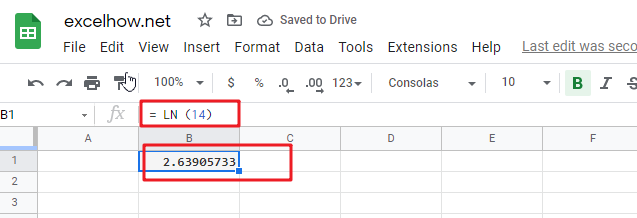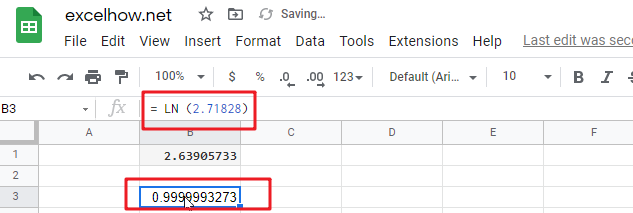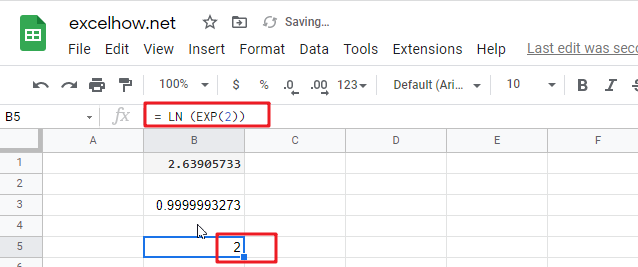# ExcelHow

This post will guide you how to use Google Sheets LN function with syntax and examples.

## Description

The Google Sheets LN function returns the natural logarithm of a given number.  And the natural logarithms are based on the constant e (the value is equal to 2.718281828…).

The purpose of this function is to get the natural logarithm of a number in google sheets. And its returned value is the natural logarithm.

The LN function is a build-in function in Google Sheets and it is categorized as a MATH function.

## Syntax

The syntax of the LN function is as below:

= LN (number)

Where the LN function arguments is:

• number – This is a required argument. The number that you want the logarithm. And the number must be a positive real number.

Note:

• If either the supplied number argument is negative or zero, the LN function will return #NUM! Error.
• If the number argument is non-numeric, the LN function will return #VALUE! Error.
• LN is the inverse of the Exp function.

## Google Sheets LN Function Examples

The below examples will show you how to use google sheets LN Function to calculate the natural logarithm of a given number.

1# to get the natural logarithm of 14, enter the following formula in Cell B1.

`= LN (14)`2# to get the natural logarithm of the value of the constant e (2.71828), enter the following formula in Cell B2.

`= LN (2.71828)`3# to get the natural logarithm of e raised to the power 2, enter the following formula in Cell B2.

= LN (EXP(2))